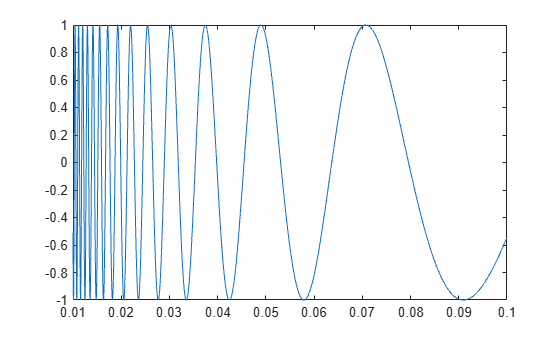Main Content

## Types of Functions

### Local and Nested Functions in a File

Program files can contain multiple functions. Local and nested functions are useful for dividing programs into smaller tasks, making it easier to read and maintain your code.

Local functions are subroutines that are available within the same file. Local functions are the most common way to break up programmatic tasks. In a function file, which contains only function definitions, local functions can appear in the file in any order after the main function in the file. In a script file, which contains commands and function definitions, local function must be at the end of the file. (Functions in scripts are supported in R2016b or later.)

For example, create a function file named `myfunction.m` that contains a main function, `myfunction`, and two local functions, `squareMe` and `doubleMe`:

```function b = myfunction(a) b = squareMe(a)+doubleMe(a); end function y = squareMe(x) y = x.^2; end function y = doubleMe(x) y = x.*2; end```

You can call the main function from the command line or another program file, although the local functions are only available to `myfunction`:

`myfunction(pi)`
```ans = 16.1528```

Nested functions are completely contained within another function. The primary difference between nested functions and local functions is that nested functions can use variables defined in parent functions without explicitly passing those variables as arguments.

Nested functions are useful when subroutines share data, such as applications that pass data between components. For example, create a function that allows you to set a value between 0 and 1 using either a slider or an editable text box. If you use nested functions for the callbacks, the slider and text box can share the value and each other’s handles without explicitly passing them:

```function myslider value = 0; f = figure; s = uicontrol(f,'Style','slider','Callback',@slider); e = uicontrol(f,'Style','edit','Callback',@edittext,... 'Position',[100,20,100,20]); function slider(obj,~) value = obj.Value; e.String = num2str(value); end function edittext(obj,~) value = str2double(obj.String); s.Value = value; end end```

### Private Functions in a Subfolder

Like local or nested functions, private functions are accessible only to functions in a specific location. However, private functions are not in the same file as the functions that can call them. Instead, they are in a subfolder named `private`. Private functions are available only to functions in the folder immediately above the `private` folder. Use private functions to separate code into different files, or to share code between multiple, related functions.

### Anonymous Functions Without a File

Anonymous functions allow you to define a function without creating a program file, as long as the function consists of a single statement. A common application of anonymous functions is to define a mathematical expression, and then evaluate that expression over a range of values using a MATLAB® function function, i.e., a function that accepts a function handle as an input.

For example, this statement creates a function handle named `s` for an anonymous function:

`s = @(x) sin(1./x);`

This function has a single input, `x`. The `@` operator creates the function handle.

You can use the function handle to evaluate the function for particular values, such as

`y = s(pi)`
```y = 0.3130 ```

Or, you can pass the function handle to a function that evaluates over a range of values, such as `fplot`:

```range = [0.01,0.1]; fplot(s,range)```Download ebook